# Concise Selina Solutions for Class 9 Maths Chapter 14- Rectilinear figures

Selina Solutions are considered to be very useful when you are preparing for the ICSE Class 9 Maths exams. Here, we bring to you detailed answers to the exercises of Selina Solutions for Class 9 Maths Chapter 14- Rectilinear figures. The chapter deals with different quadrilaterals, namely, Parallelogram, Rectangle, Rhombus, Square and Trapezium.Here, the PDF of the Class 9 maths Chapter 14 Selina solutions is available which can be downloaded as well as viewed online.

### Download PDF of Selina Solutions for Class 9 Maths Chapter 14:-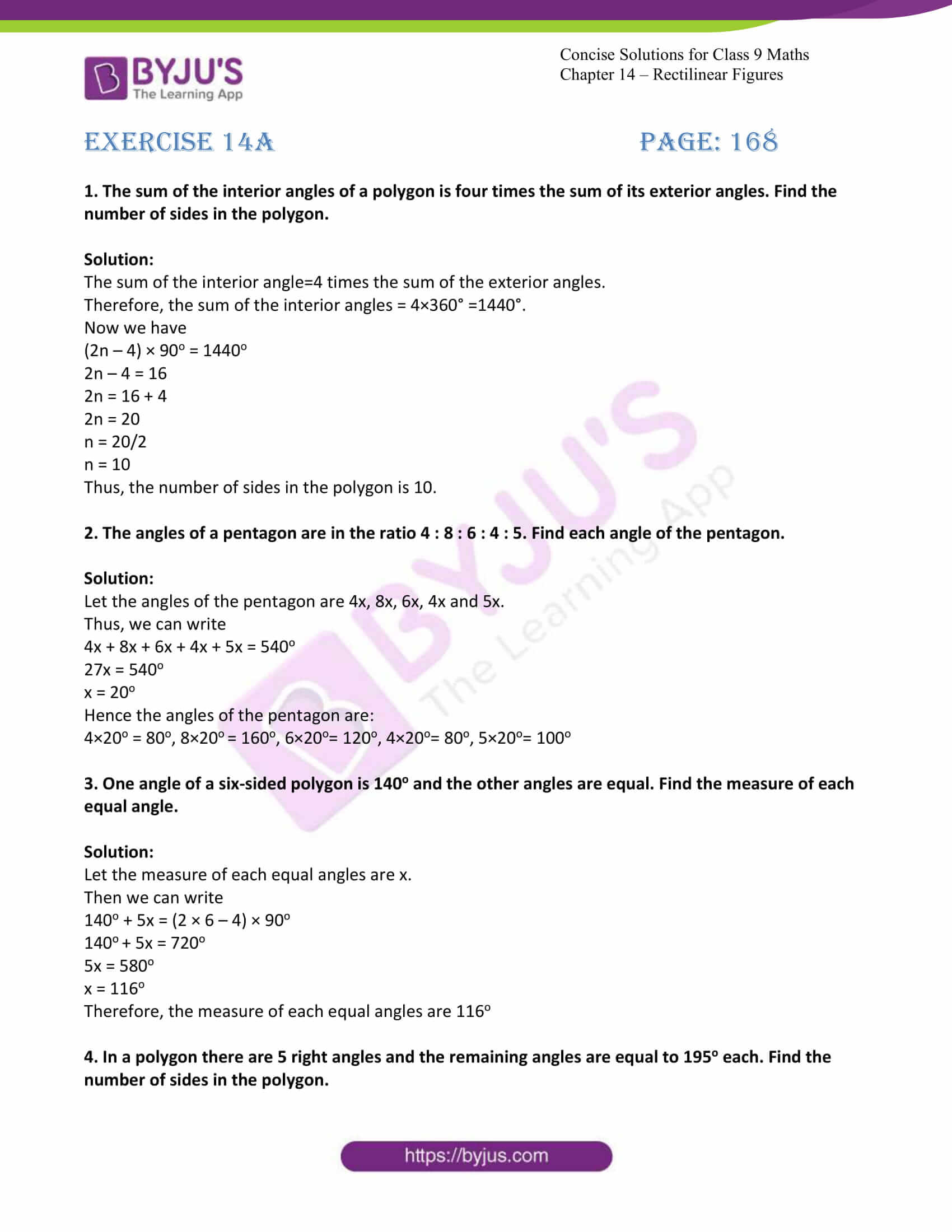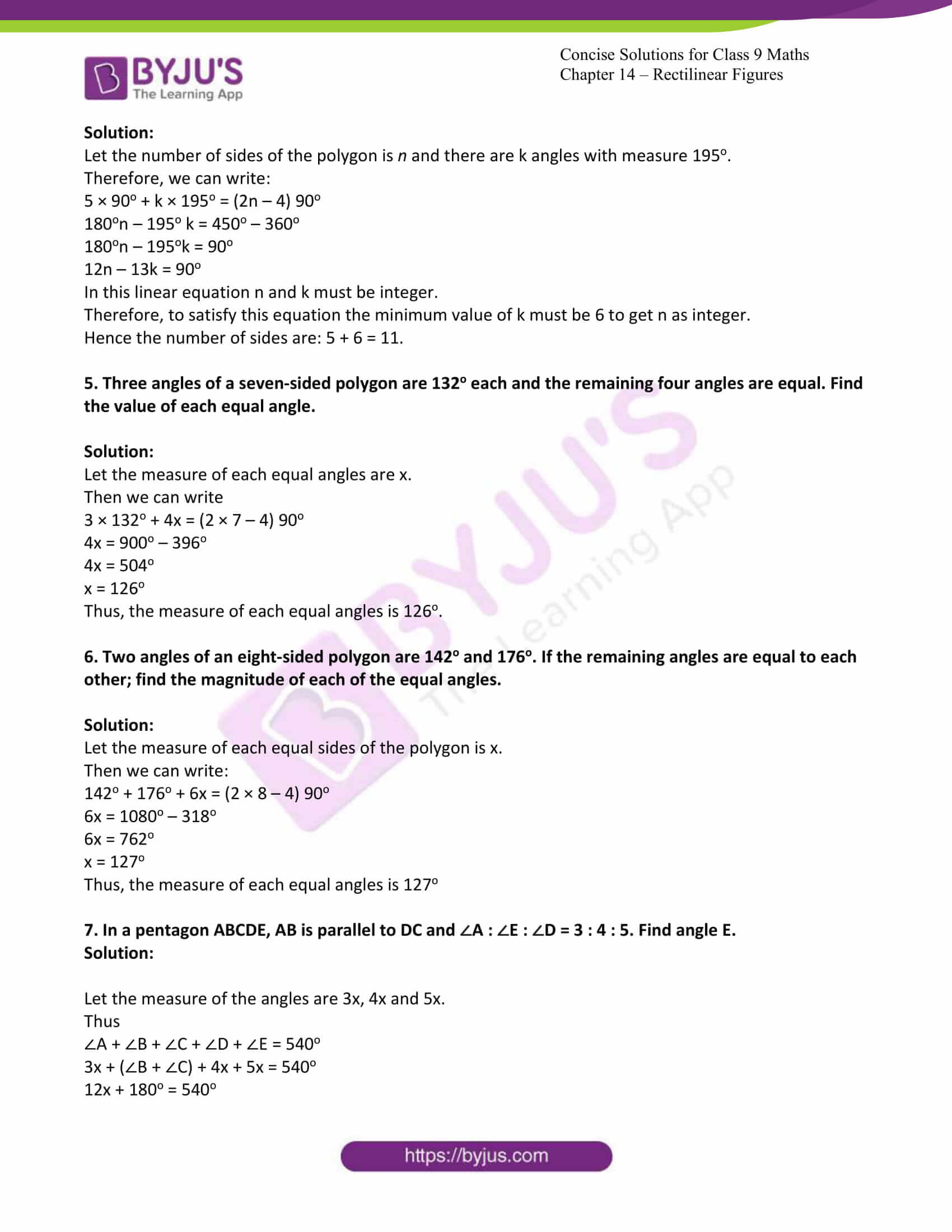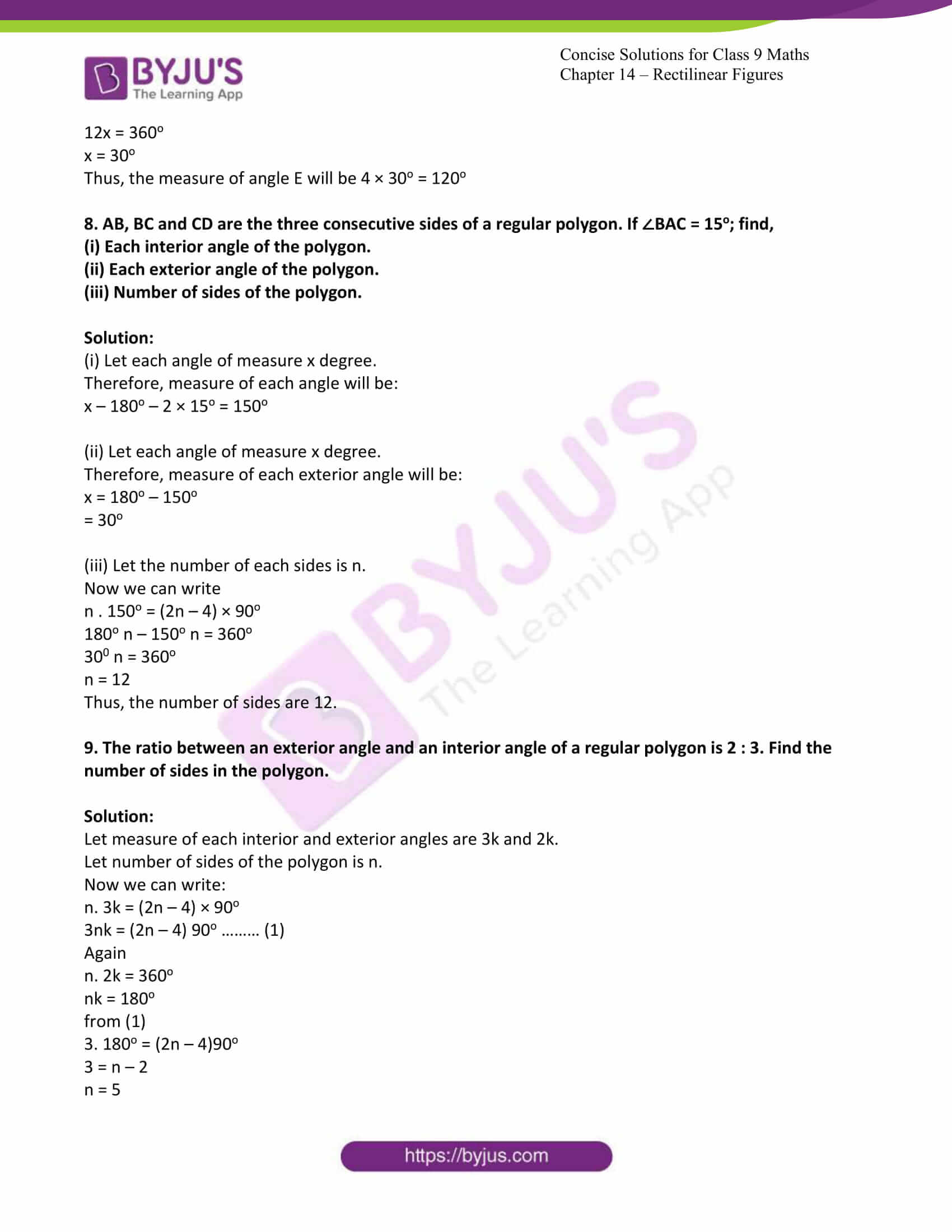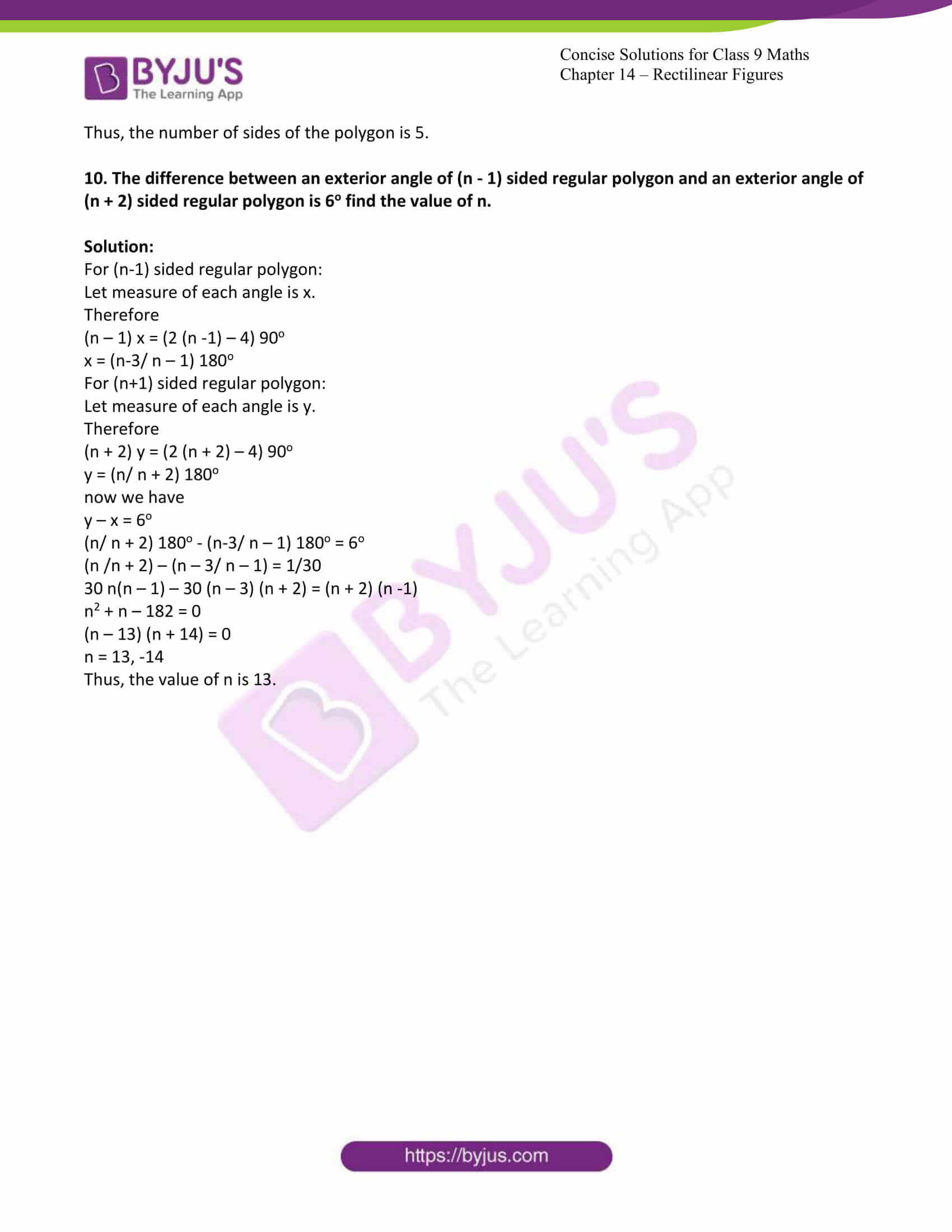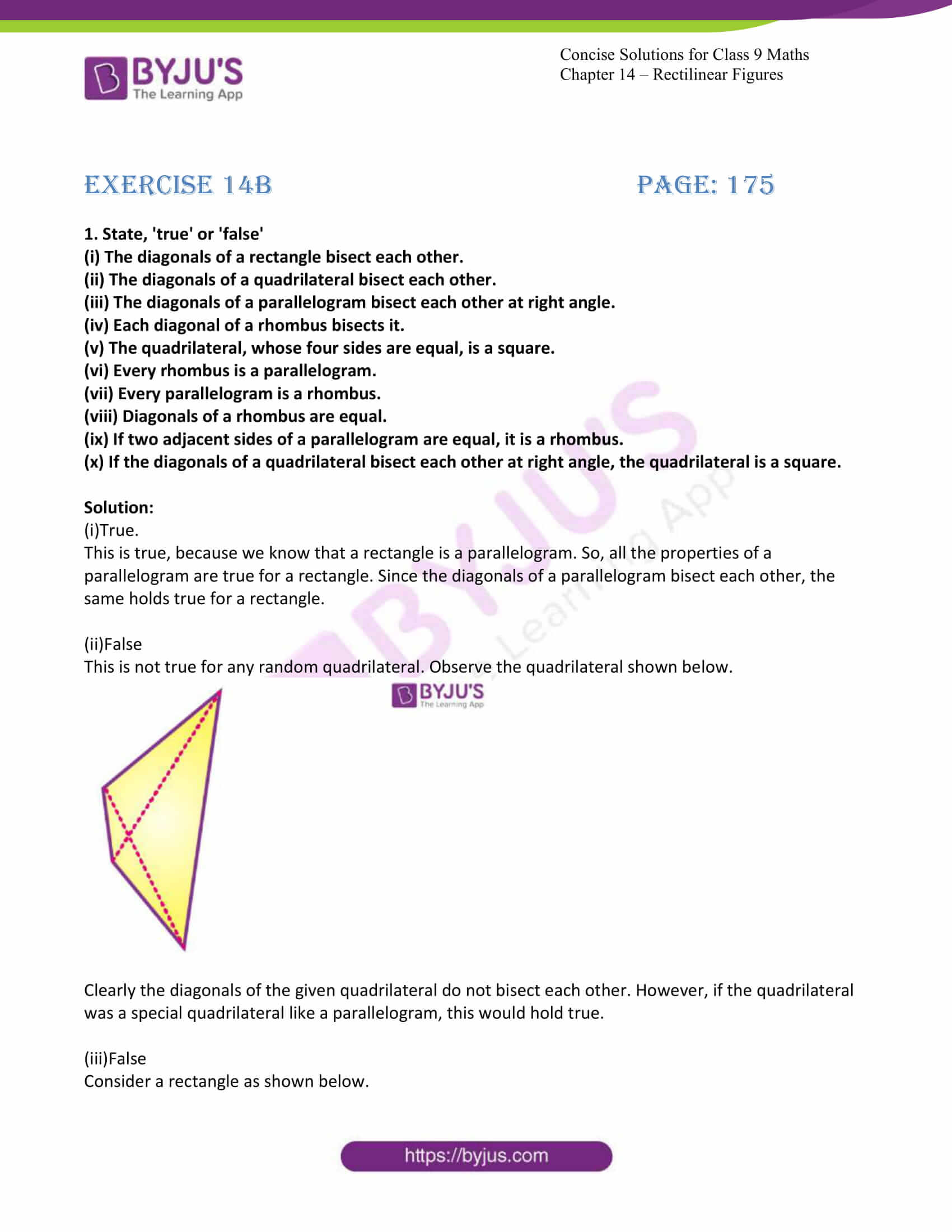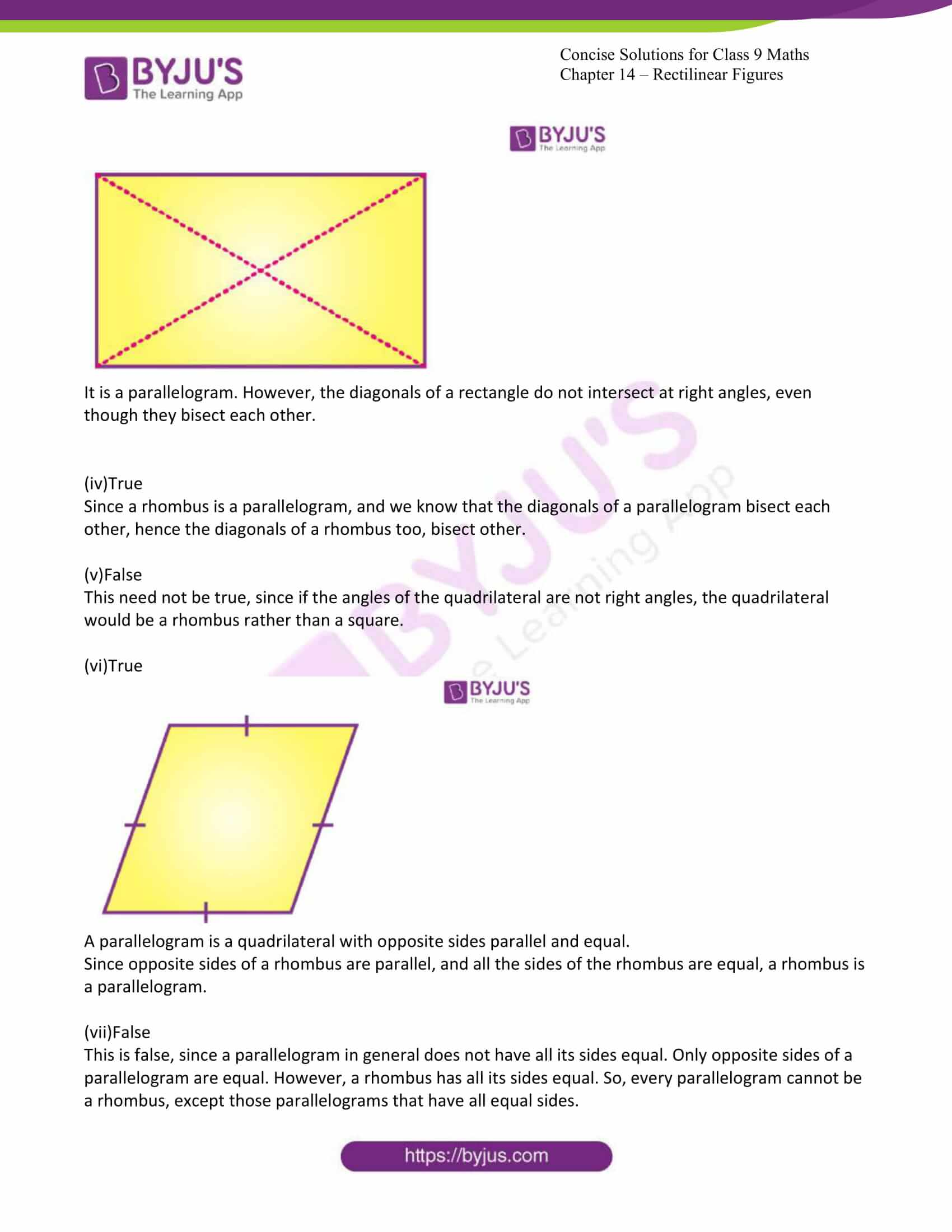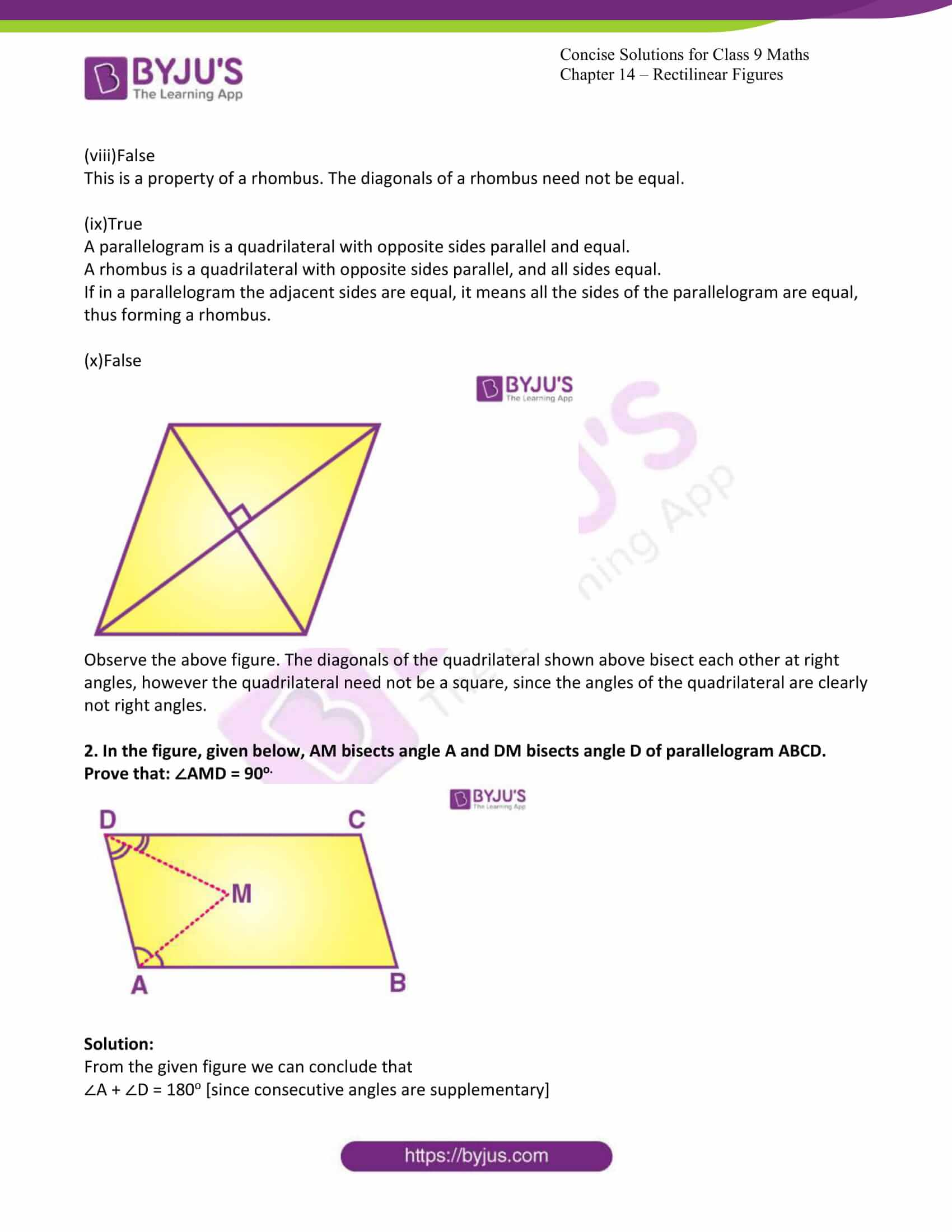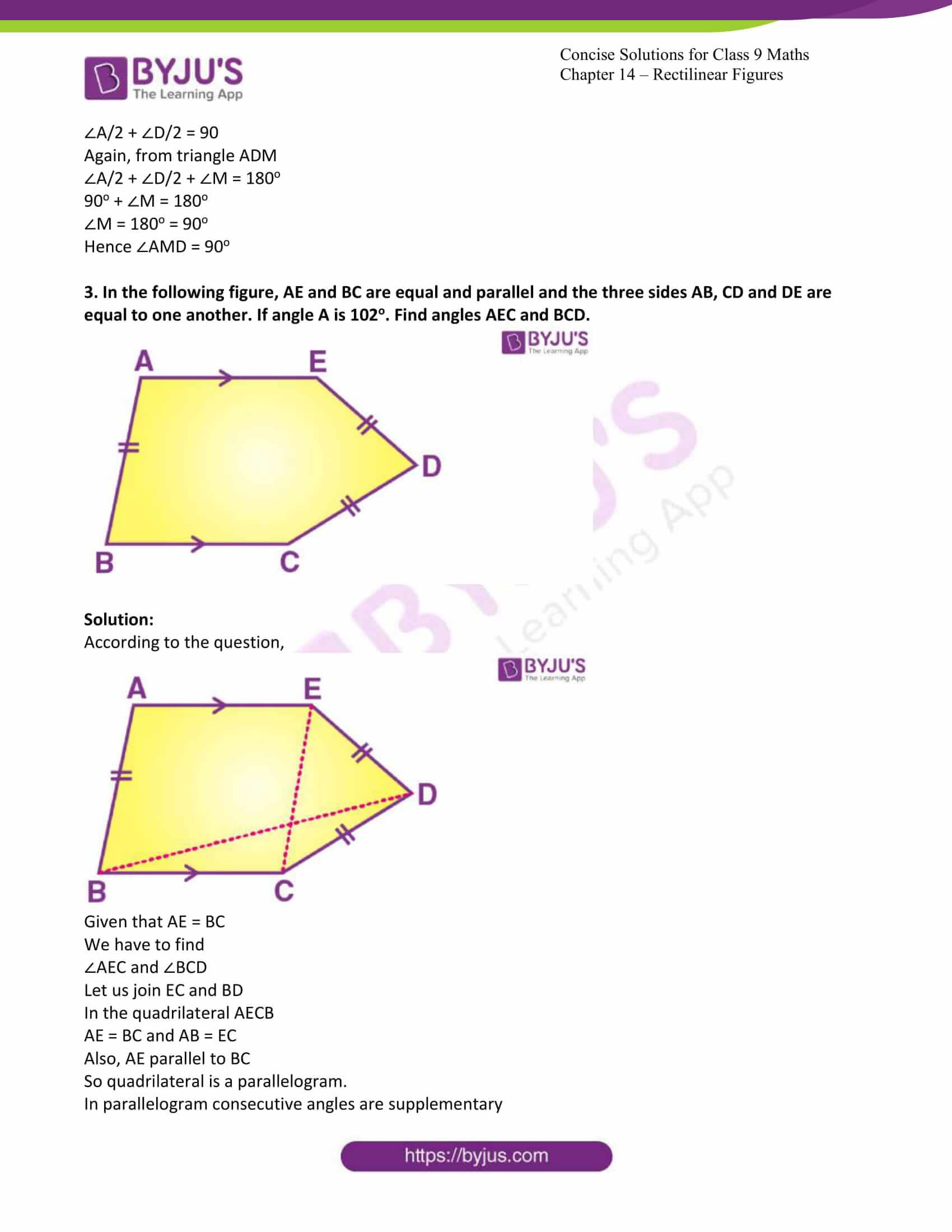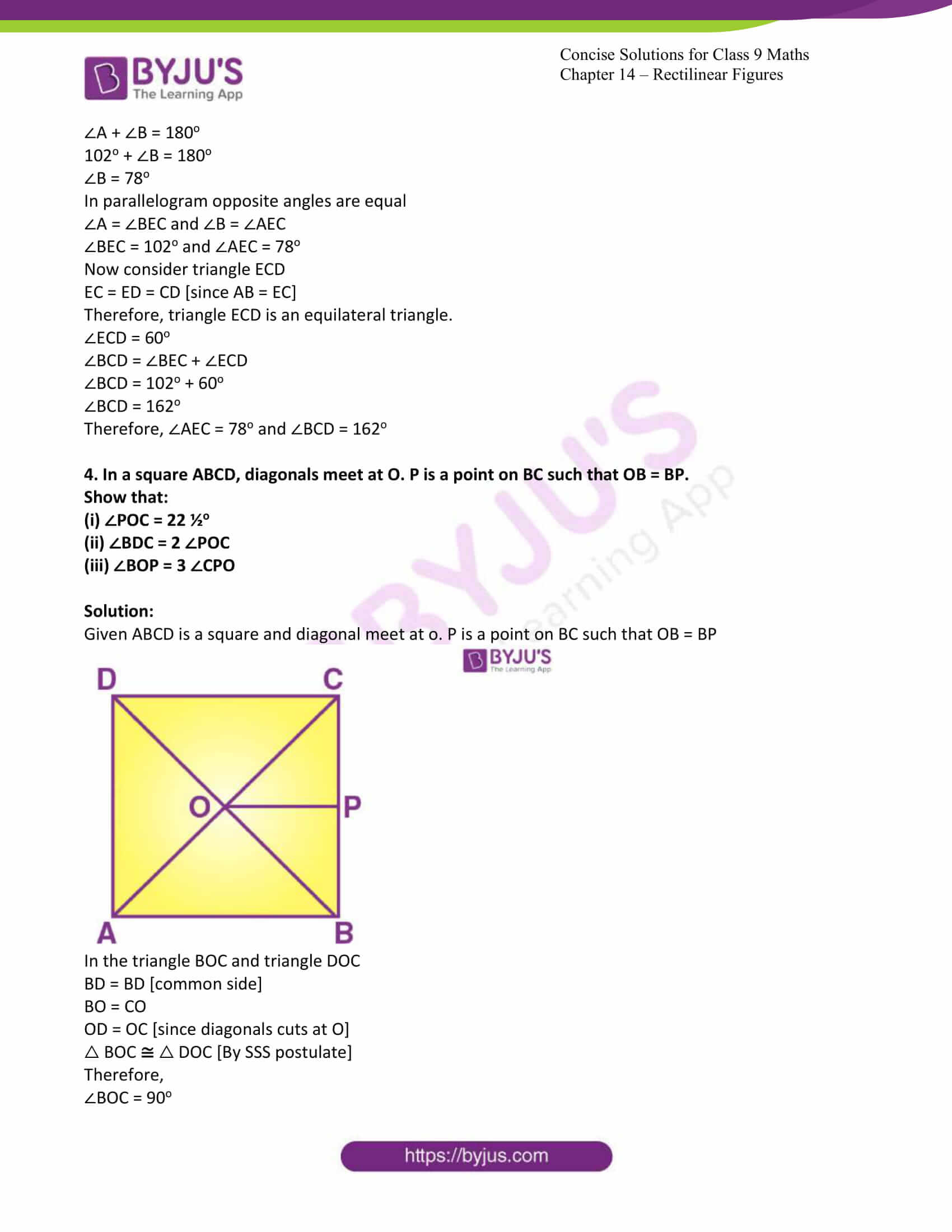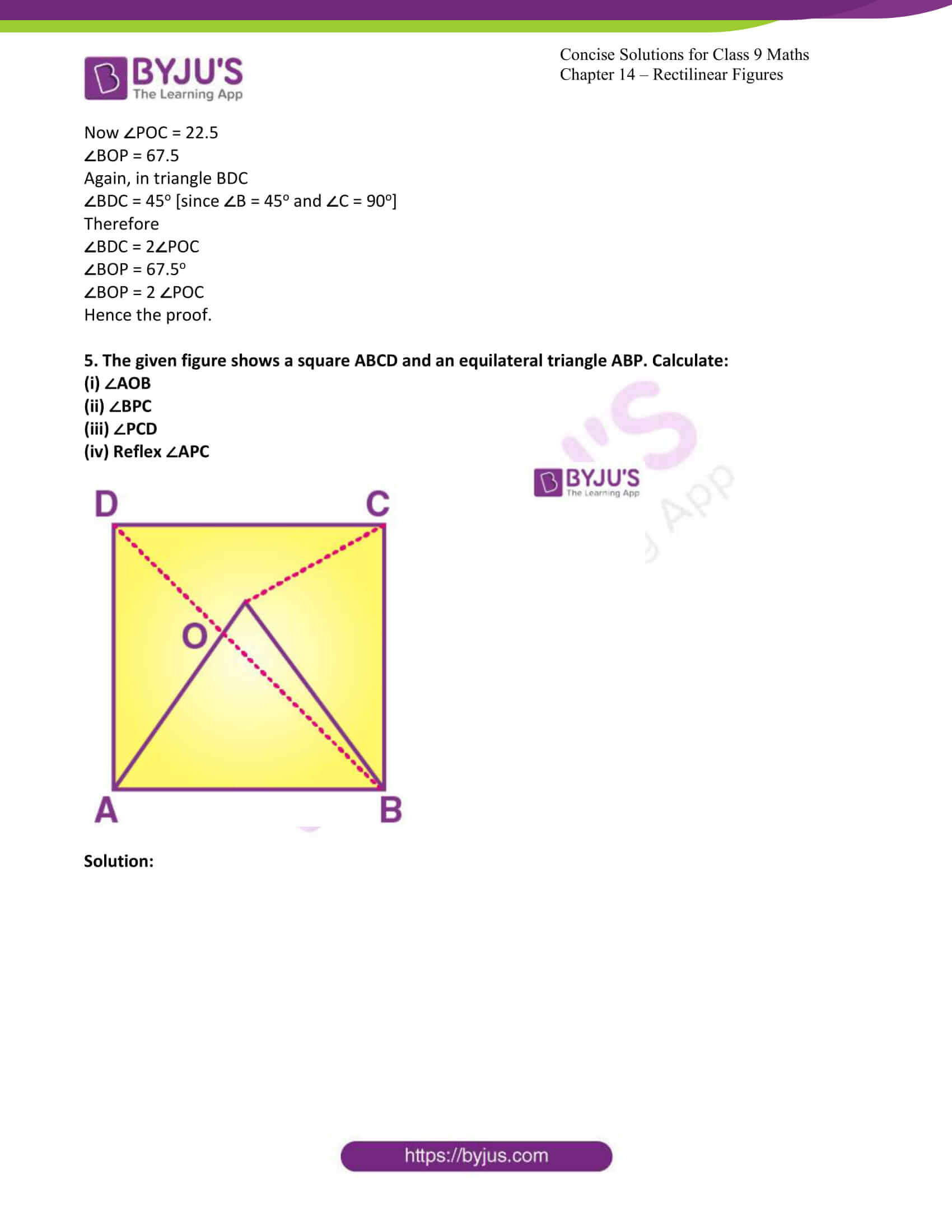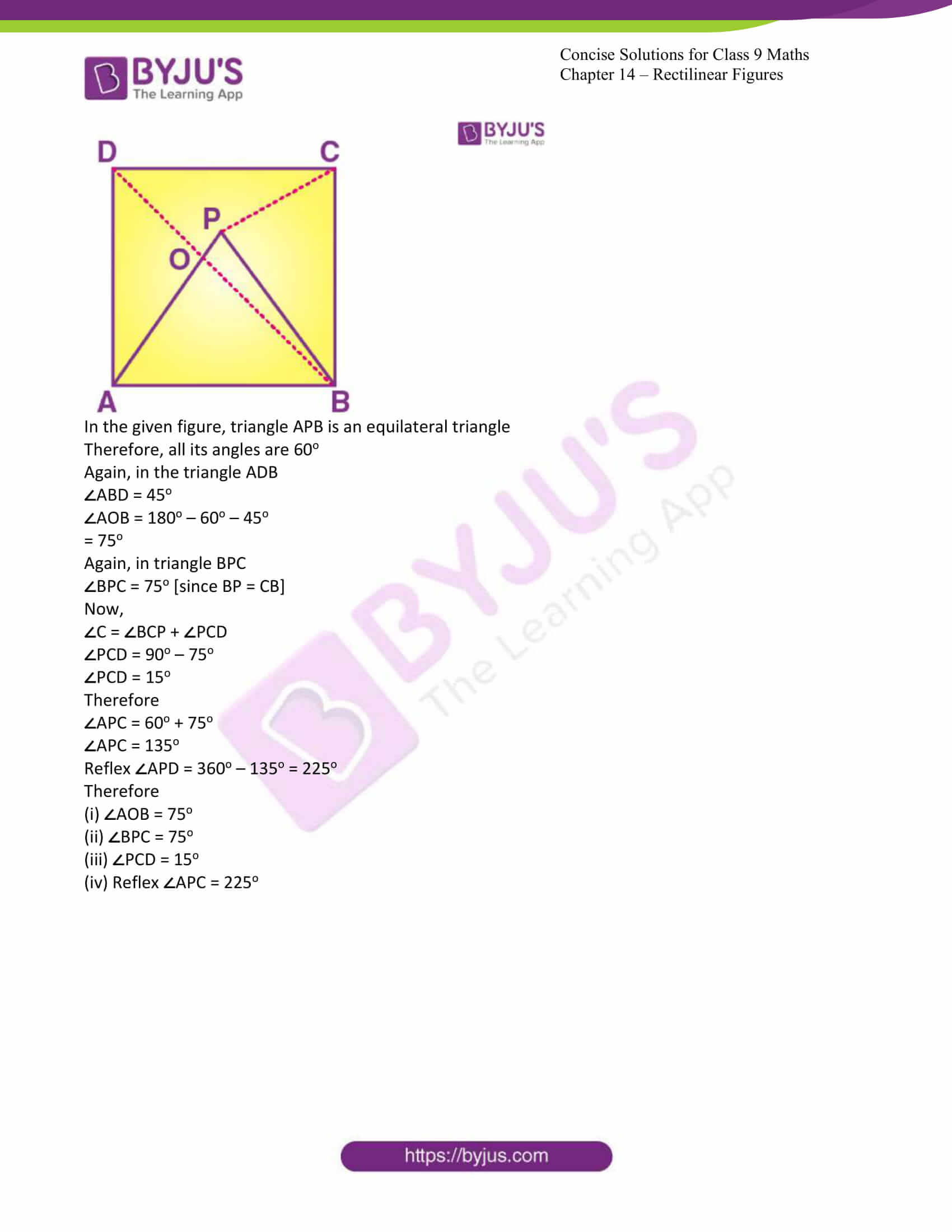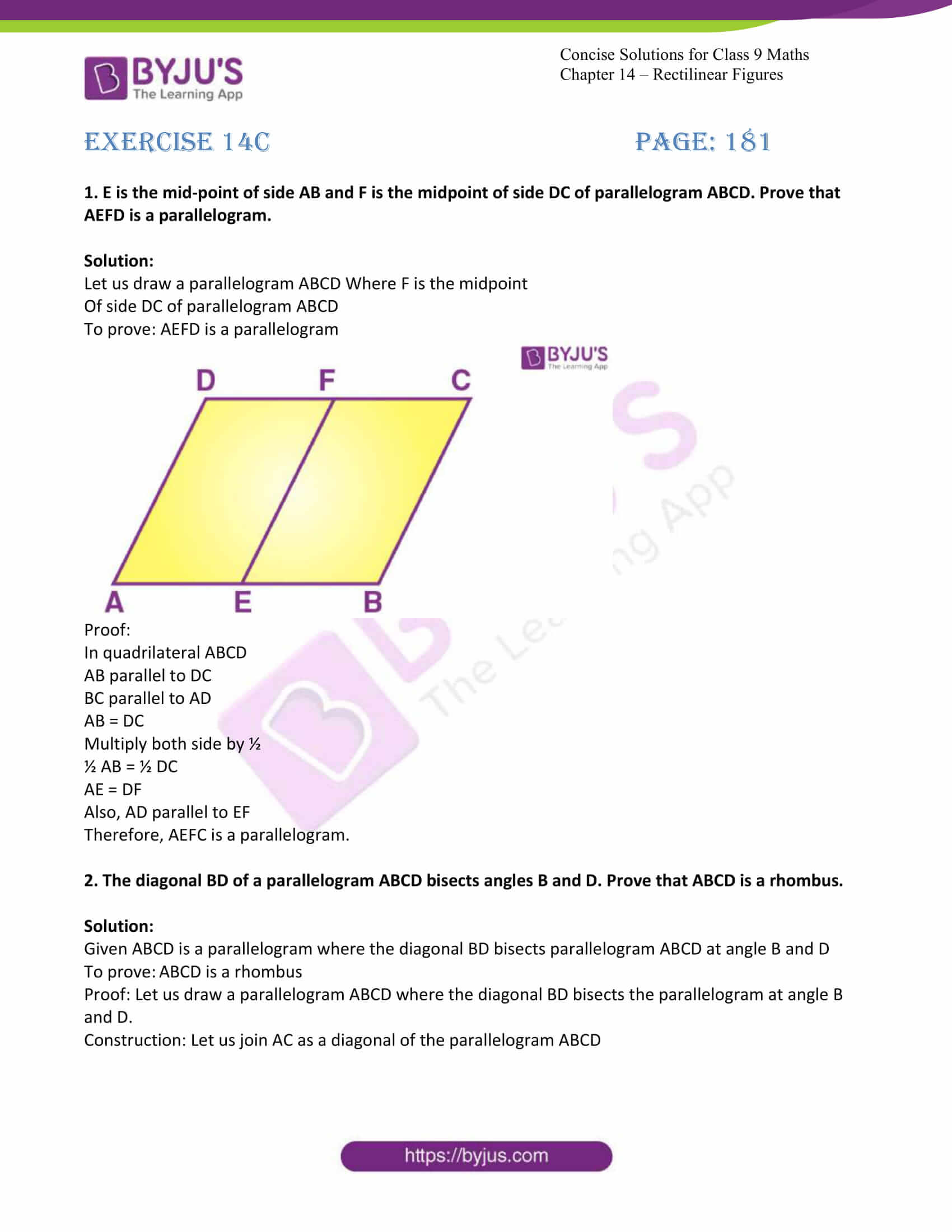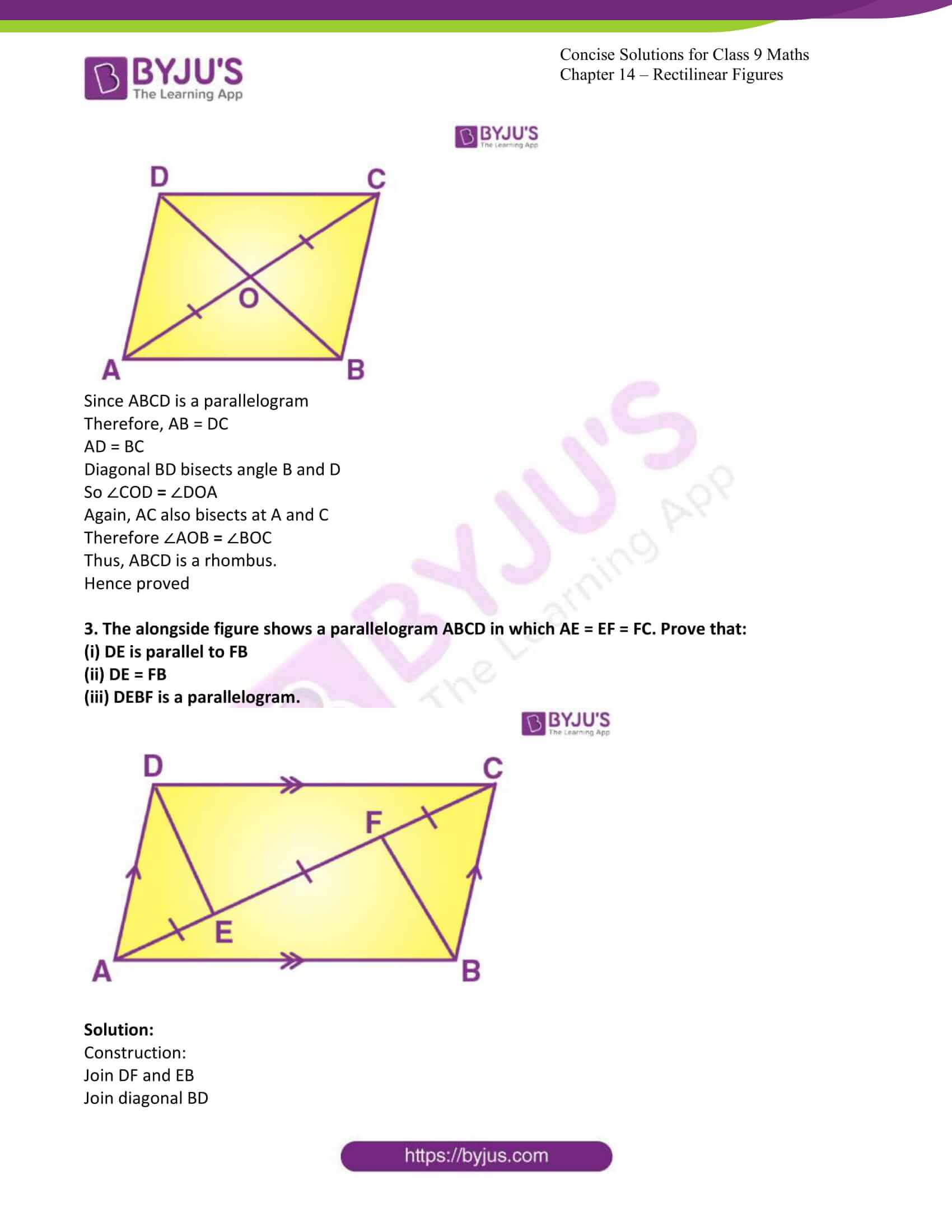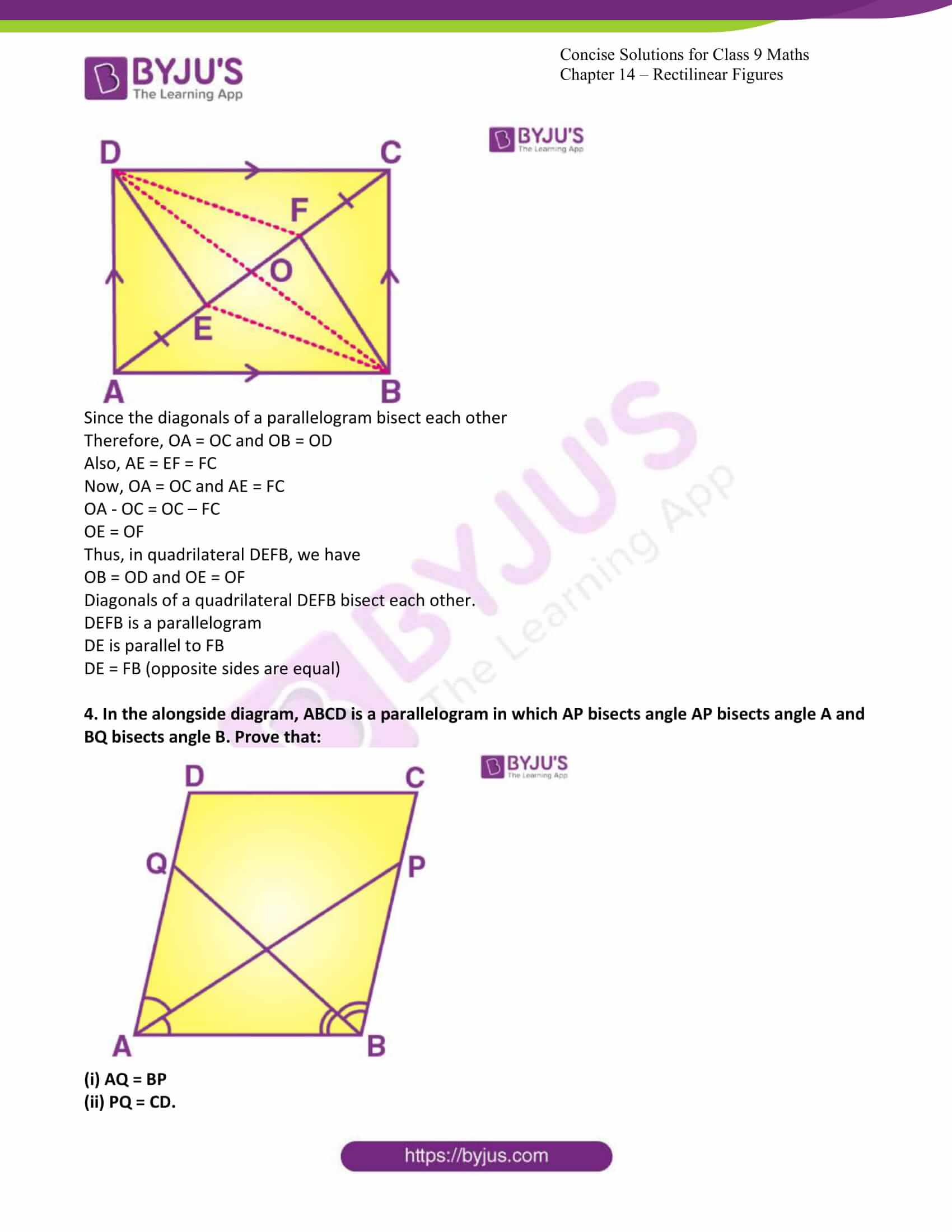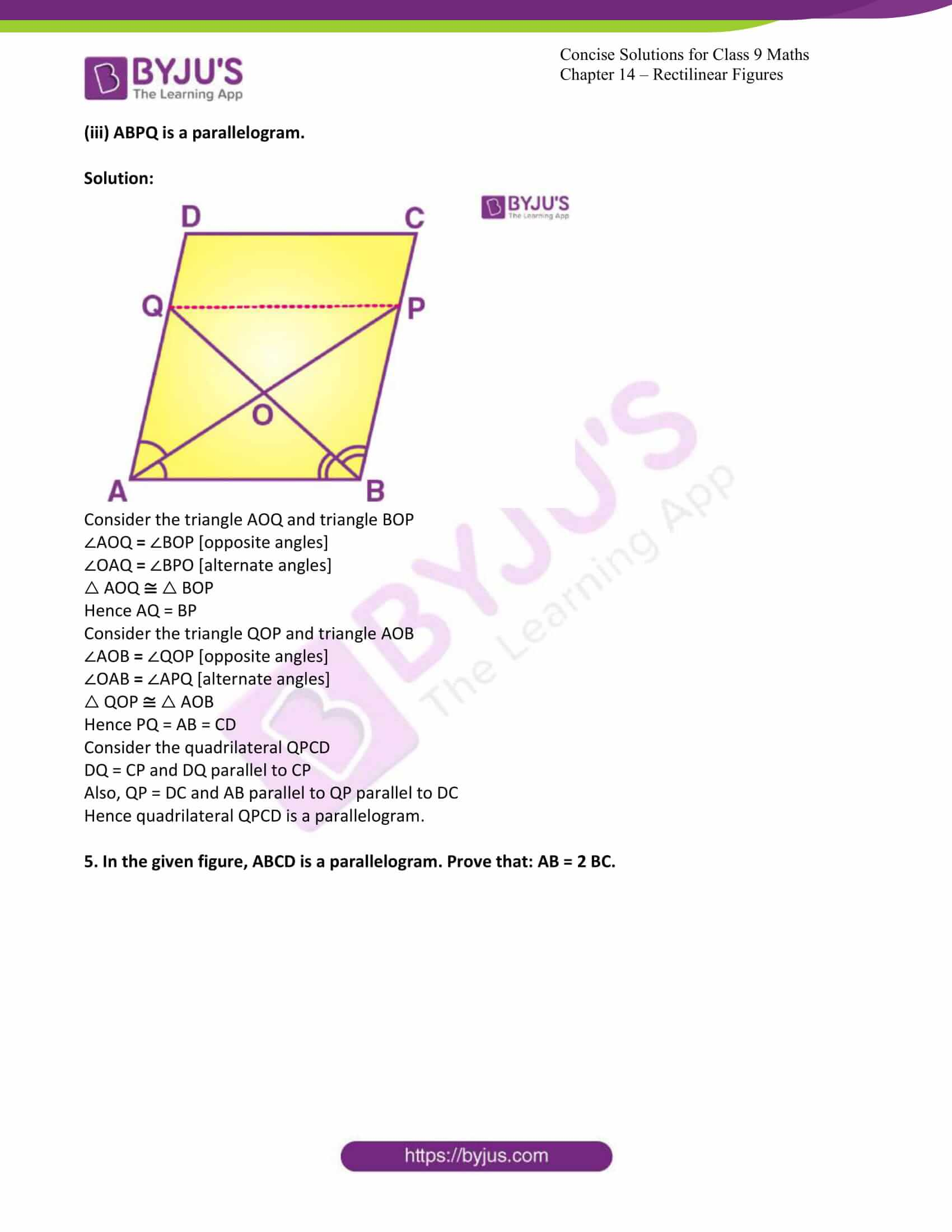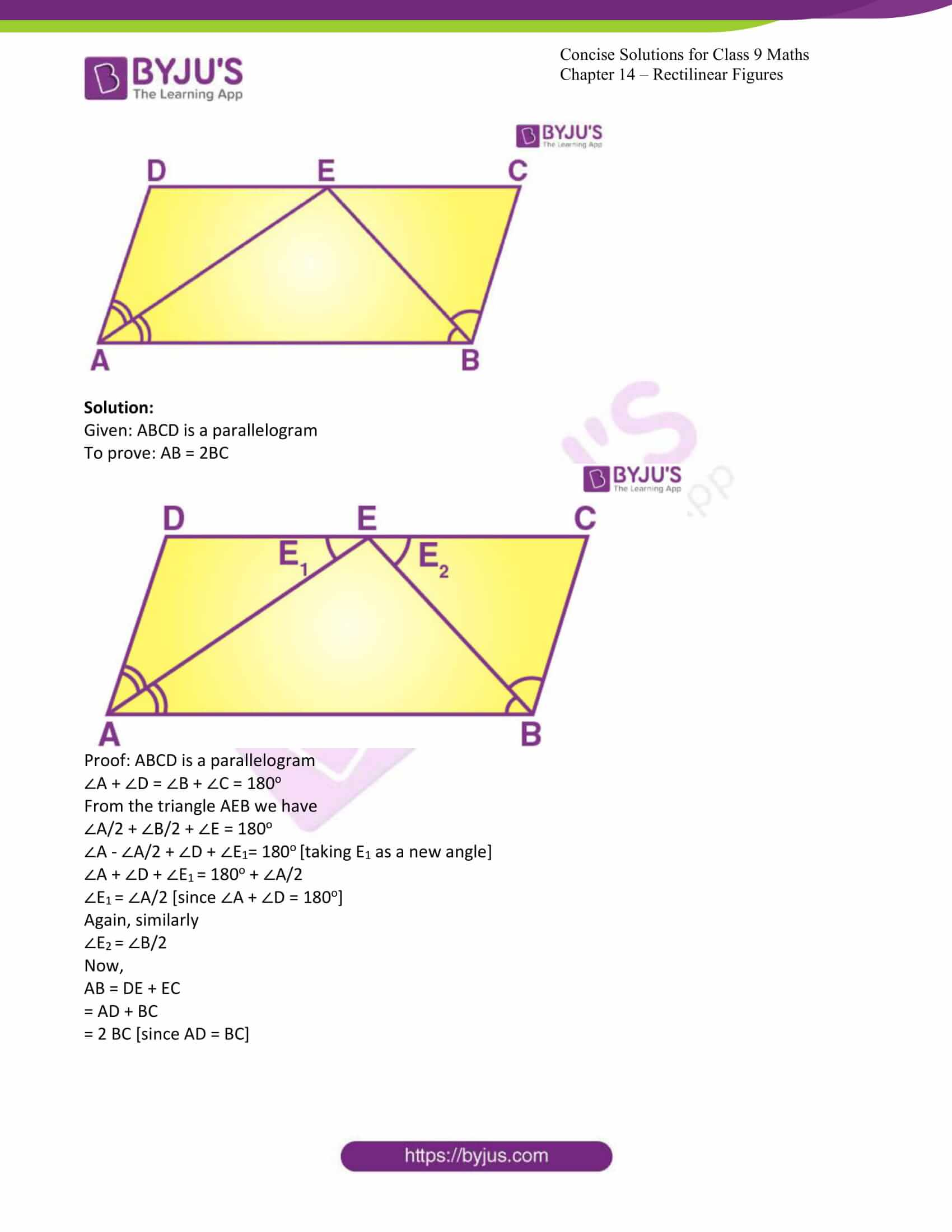Exercise 14A PAGE: 168

1. The sum of the interior angles of a polygon is four times the sum of its exterior angles. Find the number of sides in the polygon.

Solution:

The sum of the interior angle=4 times the sum of the exterior angles.

Therefore, the sum of the interior angles = 4×360° =1440°.

Now we have

(2n – 4) × 90o = 1440o

2n – 4 = 16

2n = 16 + 4

2n = 20

n = 20/2

n = 10

Thus, the number of sides in the polygon is 10.

2. The angles of a pentagon are in the ratio 4 : 8 : 6 : 4 : 5. Find each angle of the pentagon.

Solution:

Let the angles of the pentagon are 4x, 8x, 6x, 4x and 5x.

Thus, we can write

4x + 8x + 6x + 4x + 5x = 540o

27x = 540o

x = 20o

Hence the angles of the pentagon are:

4×20o = 80o, 8×20o = 160o, 6×20o= 120o, 4×20o= 80o, 5×20o= 100o

3. One angle of a six-sided polygon is 140o and the other angles are equal. Find the measure of each equal angle.

Solution:

Let the measure of each equal angles are x.

Then we can write

140o + 5x = (2 × 6 – 4) × 90o

140o + 5x = 720o

5x = 580o

x = 116o

Therefore, the measure of each equal angles are 116o

4. In a polygon there are 5 right angles and the remaining angles are equal to 195o each. Find the number of sides in the polygon.

Solution:

Let the number of sides of the polygon is n and there are k angles with measure 195o.

Therefore, we can write:

5 × 90o + k × 195o = (2n – 4) 90o

180on – 195o k = 450o – 360o

180on – 195ok = 90o

12n – 13k = 90o

In this linear equation n and k must be integer.

Therefore, to satisfy this equation the minimum value of k must be 6 to get n as integer.

Hence the number of sides are: 5 + 6 = 11.

5. Three angles of a seven-sided polygon are 132o each and the remaining four angles are equal. Find the value of each equal angle.

Solution:

Let the measure of each equal angles are x.

Then we can write

3 × 132o + 4x = (2 × 7 – 4) 90o

4x = 900o – 396o

4x = 504o

x = 126o

Thus, the measure of each equal angles is 126o.

6. Two angles of an eight-sided polygon are 142o and 176o. If the remaining angles are equal to each other; find the magnitude of each of the equal angles.

Solution:

Let the measure of each equal sides of the polygon is x.

Then we can write:

142o + 176o + 6x = (2 × 8 – 4) 90o

6x = 1080o – 318o

6x = 762o

x = 127o

Thus, the measure of each equal angles is 127o

7. In a pentagon ABCDE, AB is parallel to DC and ∠A : ∠E : ∠D = 3 : 4 : 5. Find angle E.

Solution:

Let the measure of the angles are 3x, 4x and 5x.

Thus

∠A + ∠B + ∠C + ∠D + ∠E = 540o

3x + (∠B + ∠C) + 4x + 5x = 540o

12x + 180o = 540o

12x = 360o

x = 30o

Thus, the measure of angle E will be 4 × 30o = 120o

8. AB, BC and CD are the three consecutive sides of a regular polygon. If ∠BAC = 15o; find,

(i) Each interior angle of the polygon.

(ii) Each exterior angle of the polygon.

(iii) Number of sides of the polygon.

Solution:

(i) Let each angle of measure x degree.

Therefore, measure of each angle will be:

x – 180o – 2 × 15o = 150o

(ii) Let each angle of measure x degree.

Therefore, measure of each exterior angle will be:

x = 180o – 150o

= 30o

(iii) Let the number of each sides is n.

Now we can write

n . 150o = (2n – 4) × 90o

180o n – 150o n = 360o

300 n = 360o

n = 12

Thus, the number of sides are 12.

9. The ratio between an exterior angle and an interior angle of a regular polygon is 2 : 3. Find the number of sides in the polygon.

Solution:

Let measure of each interior and exterior angles are 3k and 2k.

Let number of sides of the polygon is n.

Now we can write:

n. 3k = (2n – 4) × 90o

3nk = (2n – 4) 90o ……… (1)

Again

n. 2k = 360o

nk = 180o

from (1)

3. 180o = (2n – 4)90o

3 = n – 2

n = 5

Thus, the number of sides of the polygon is 5.

10. The difference between an exterior angle of (n – 1) sided regular polygon and an exterior angle of (n + 2) sided regular polygon is 6o find the value of n.

Solution:

For (n-1) sided regular polygon:

Let measure of each angle is x.

Therefore

(n – 1) x = (2 (n -1) – 4) 90o

x = (n-3/ n – 1) 180o

For (n+1) sided regular polygon:

Let measure of each angle is y.

Therefore

(n + 2) y = (2 (n + 2) – 4) 90o

y = (n/ n + 2) 180o

now we have

y – x = 6o

(n/ n + 2) 180o – (n-3/ n – 1) 180o = 6o

(n /n + 2) – (n – 3/ n – 1) = 1/30

30 n(n – 1) – 30 (n – 3) (n + 2) = (n + 2) (n -1)

n2 + n – 182 = 0

(n – 13) (n + 14) = 0

n = 13, -14

Thus, the value of n is 13.

Exercise 14B PAGE: 175

1. State, ‘true’ or ‘false’

(i) The diagonals of a rectangle bisect each other.

(ii) The diagonals of a quadrilateral bisect each other.

(iii) The diagonals of a parallelogram bisect each other at right angle.

(iv) Each diagonal of a rhombus bisects it.

(v) The quadrilateral, whose four sides are equal, is a square.

(vi) Every rhombus is a parallelogram.

(vii) Every parallelogram is a rhombus.

(viii) Diagonals of a rhombus are equal.

(ix) If two adjacent sides of a parallelogram are equal, it is a rhombus.

(x) If the diagonals of a quadrilateral bisect each other at right angle, the quadrilateral is a square.

Solution:

(i)True.

This is true, because we know that a rectangle is a parallelogram. So, all the properties of a parallelogram are true for a rectangle. Since the diagonals of a parallelogram bisect each other, the same holds true for a rectangle.

(ii)False

This is not true for any random quadrilateral. Observe the quadrilateral shown below.Clearly the diagonals of the given quadrilateral do not bisect each other. However, if the quadrilateral was a special quadrilateral like a parallelogram, this would hold true.

(iii)False

Consider a rectangle as shown below.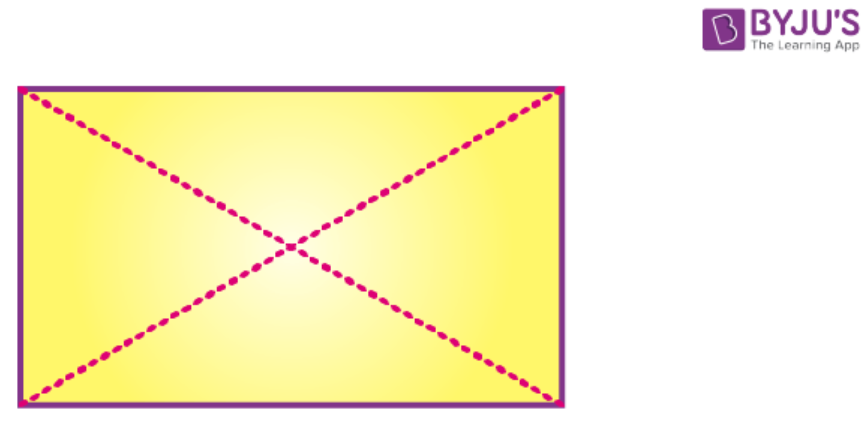It is a parallelogram. However, the diagonals of a rectangle do not intersect at right angles, even though they bisect each other.

(iv)True

Since a rhombus is a parallelogram, and we know that the diagonals of a parallelogram bisect each other, hence the diagonals of a rhombus too, bisect other.

(v)False

This need not be true, since if the angles of the quadrilateral are not right angles, the quadrilateral would be a rhombus rather than a square.

(vi)True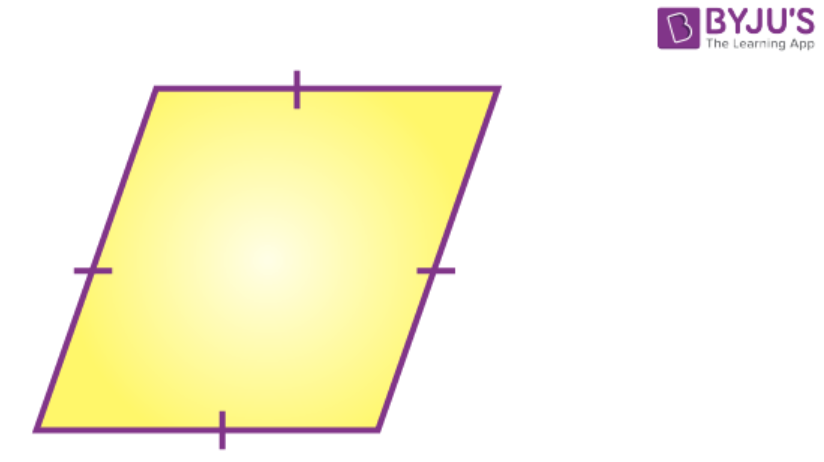A parallelogram is a quadrilateral with opposite sides parallel and equal.

Since opposite sides of a rhombus are parallel, and all the sides of the rhombus are equal, a rhombus is a parallelogram.

(vii)False

This is false, since a parallelogram in general does not have all its sides equal. Only opposite sides of a parallelogram are equal. However, a rhombus has all its sides equal. So, every parallelogram cannot be a rhombus, except those parallelograms that have all equal sides.

(viii)False

This is a property of a rhombus. The diagonals of a rhombus need not be equal.

(ix)True

A parallelogram is a quadrilateral with opposite sides parallel and equal.

A rhombus is a quadrilateral with opposite sides parallel, and all sides equal.

If in a parallelogram the adjacent sides are equal, it means all the sides of the parallelogram are equal, thus forming a rhombus.

(x)FalseObserve the above figure. The diagonals of the quadrilateral shown above bisect each other at right angles, however the quadrilateral need not be a square, since the angles of the quadrilateral are clearly not right angles.

2. In the figure, given below, AM bisects angle A and DM bisects angle D of parallelogram ABCD. Prove that: ∠AMD = 90o.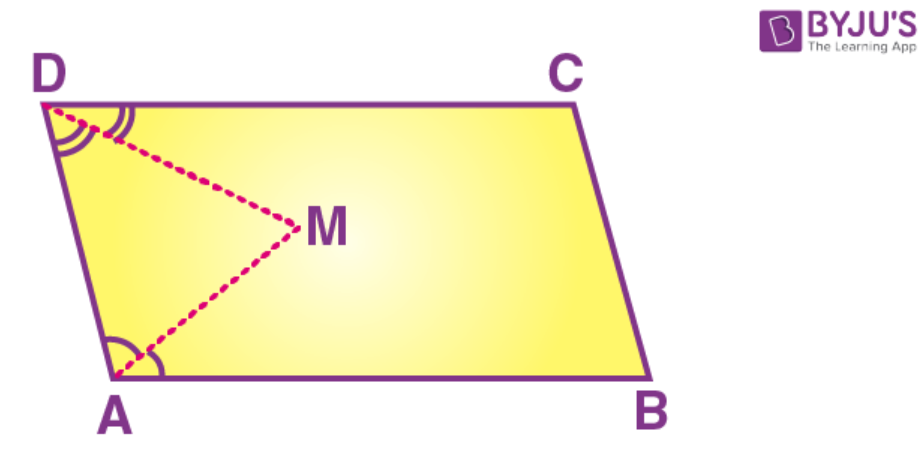Solution:

From the given figure we can conclude that

∠A + ∠D = 180o [since consecutive angles are supplementary]

∠A/2 + ∠D/2 = 90

∠A/2 + ∠D/2 + ∠M = 180o

90o + ∠M = 180o

∠M = 180o = 90o

Hence ∠AMD = 90o

3. In the following figure, AE and BC are equal and parallel and the three sides AB, CD and DE are equal to one another. If angle A is 102o. Find angles AEC and BCD.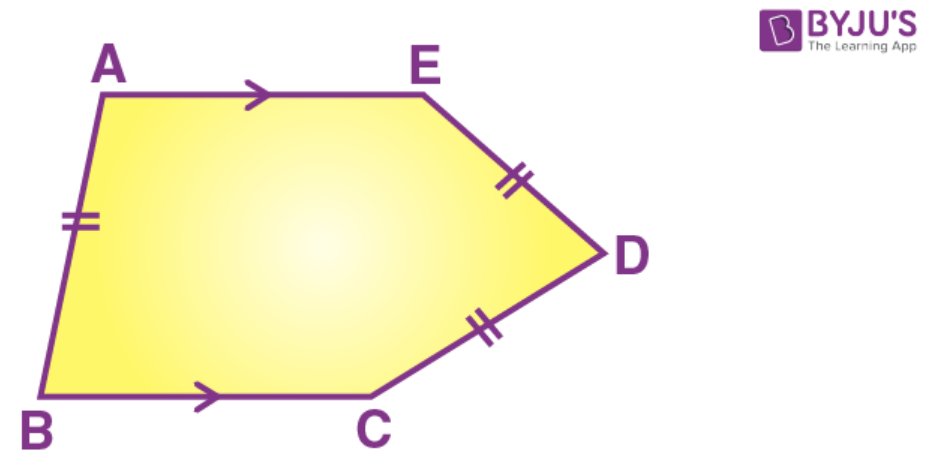Solution:

According to the question,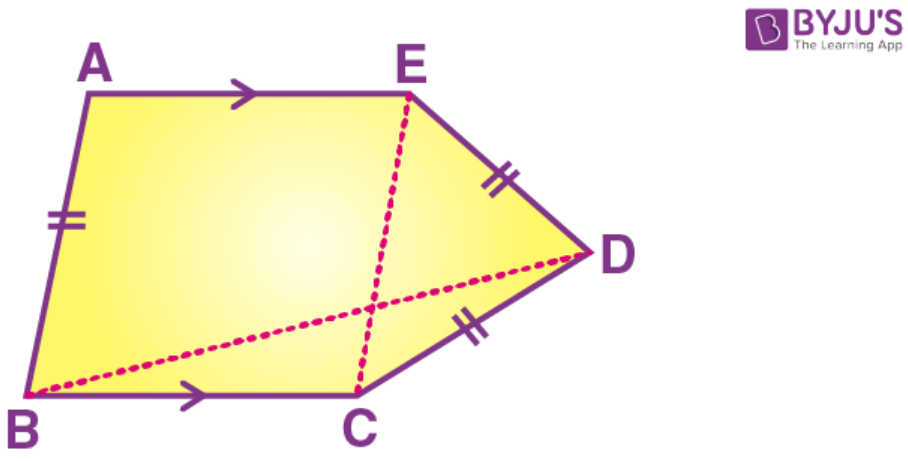Given that AE = BC

We have to find

∠AEC and ∠BCD

Let us join EC and BD

AE = BC and AB = EC

Also, AE parallel to BC

In parallelogram consecutive angles are supplementary

∠A + ∠B = 180o

102o + ∠B = 180o

∠B = 78o

In parallelogram opposite angles are equal

∠A = ∠BEC and ∠B = ∠AEC

∠BEC = 102o and ∠AEC = 78o

Now consider triangle ECD

EC = ED = CD [since AB = EC]

Therefore, triangle ECD is an equilateral triangle.

∠ECD = 60o

∠BCD = ∠BEC + ∠ECD

∠BCD = 102o + 60o

∠BCD = 162o

Therefore, ∠AEC = 78o and ∠BCD = 162o

4. In a square ABCD, diagonals meet at O. P is a point on BC such that OB = BP.

Show that:

(i) ∠POC = 22 ½o

(ii) ∠BDC = 2 ∠POC

(iii) ∠BOP = 3 ∠CPO

Solution:

Given ABCD is a square and diagonal meet at o. P is a point on BC such that OB = BP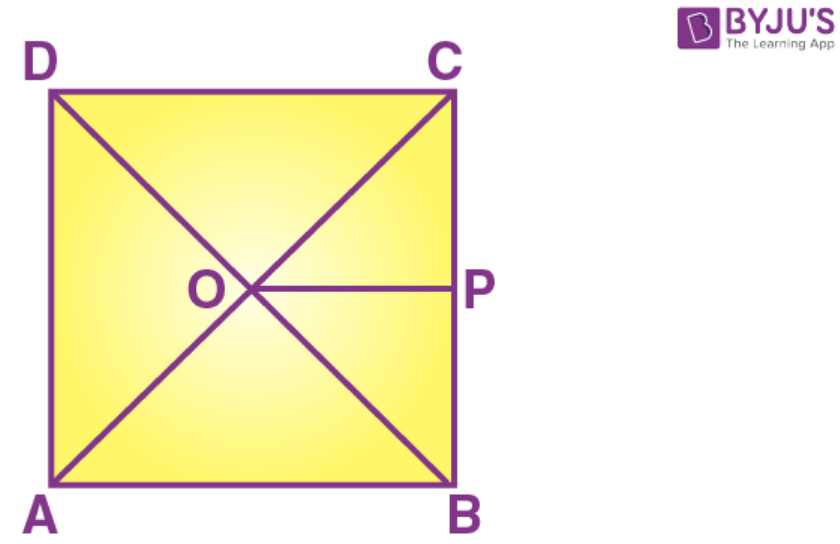In the triangle BOC and triangle DOC

BD = BD [common side]

BO = CO

OD = OC [since diagonals cuts at O]

△ BOC △ DOC [By SSS postulate]

Therefore,

∠BOC = 90o

Now POC = 22.5

BOP = 67.5

Again, in triangle BDC

BDC = 45o [since B = 45o and C = 90o]

Therefore

BDC = 2POC

BOP = 67.5o

BOP = 2 POC

Hence the proof.

5. The given figure shows a square ABCD and an equilateral triangle ABP. Calculate:

(i) ∠AOB

(ii) ∠BPC

(iii) ∠PCD

(iv) Reflex ∠APC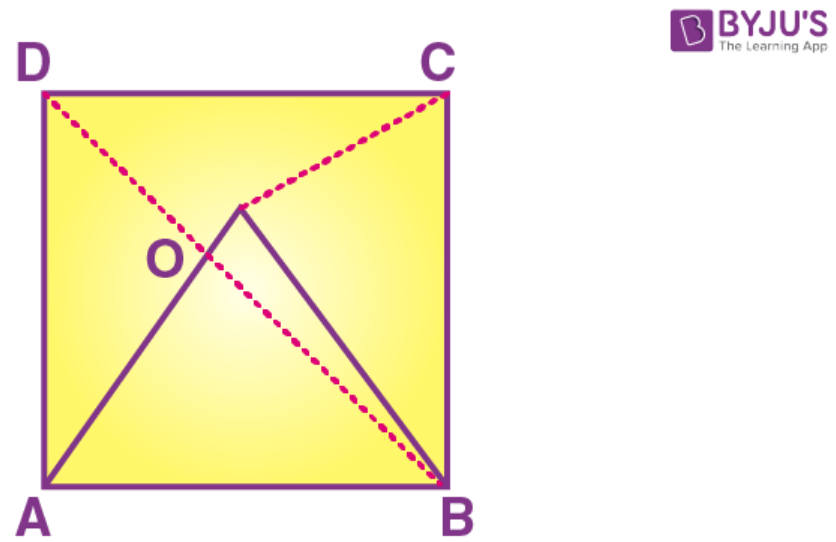Solution: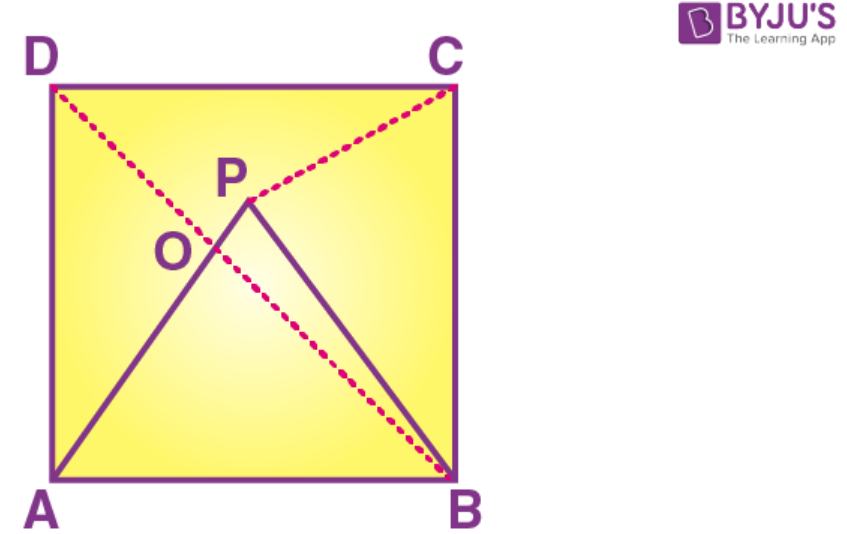In the given figure, triangle APB is an equilateral triangle

Therefore, all its angles are 60o

ABD = 45o

AOB = 180o – 60o – 45o

= 75o

Again, in triangle BPC

BPC = 75o [since BP = CB]

Now,

C = BCP + PCD

PCD = 90o – 75o

PCD = 15o

Therefore

APC = 60o + 75o

APC = 135o

Reflex APD = 360o – 135o = 225o

Therefore

(i) AOB = 75o

(ii) BPC = 75o

(iii) PCD = 15o

(iv) Reflex APC = 225o

Exercise 14c PAGE: 181

1. E is the mid-point of side AB and F is the midpoint of side DC of parallelogram ABCD. Prove that AEFD is a parallelogram.

Solution:

Let us draw a parallelogram ABCD Where F is the midpoint

Of side DC of parallelogram ABCD

To prove: AEFD is a parallelogram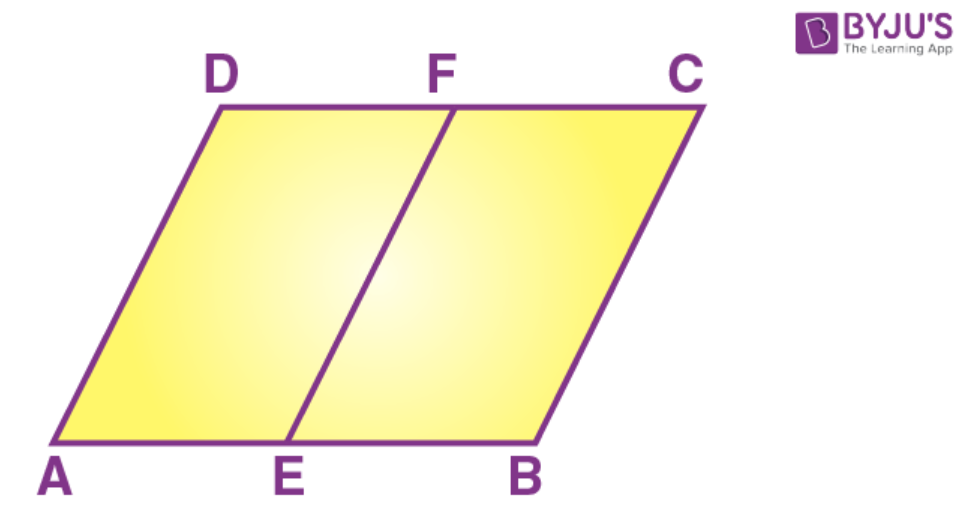Proof:

AB parallel to DC

AB = DC

Multiply both side by ½

½ AB = ½ DC

AE = DF

Therefore, AEFC is a parallelogram.

2. The diagonal BD of a parallelogram ABCD bisects angles B and D. Prove that ABCD is a rhombus.

Solution:

Given ABCD is a parallelogram where the diagonal BD bisects parallelogram ABCD at angle B and D

To prove: ABCD is a rhombus

Proof: Let us draw a parallelogram ABCD where the diagonal BD bisects the parallelogram at angle B and D.

Construction: Let us join AC as a diagonal of the parallelogram ABCD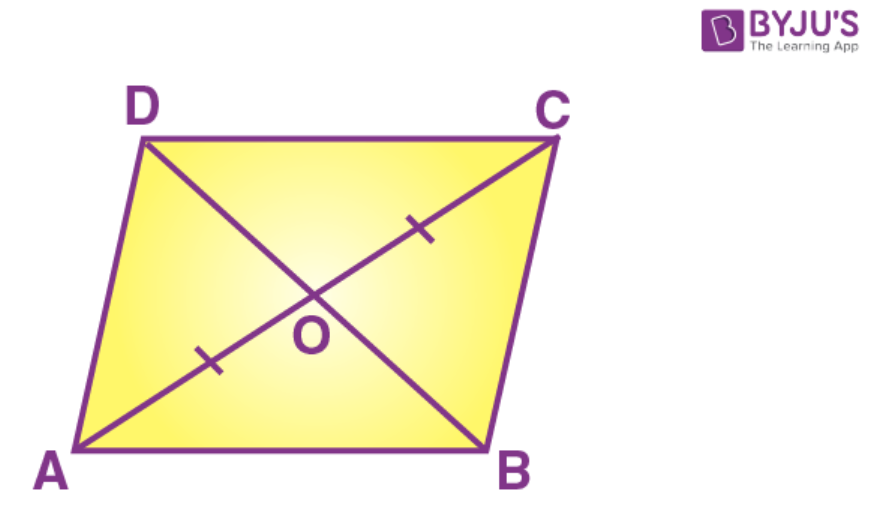Since ABCD is a parallelogram

Therefore, AB = DC

Diagonal BD bisects angle B and D

So ∠COD = ∠DOA

Again, AC also bisects at A and C

Therefore ∠AOB = ∠BOC

Thus, ABCD is a rhombus.

Hence proved

3. The alongside figure shows a parallelogram ABCD in which AE = EF = FC. Prove that:

(i) DE is parallel to FB

(ii) DE = FB

(iii) DEBF is a parallelogram.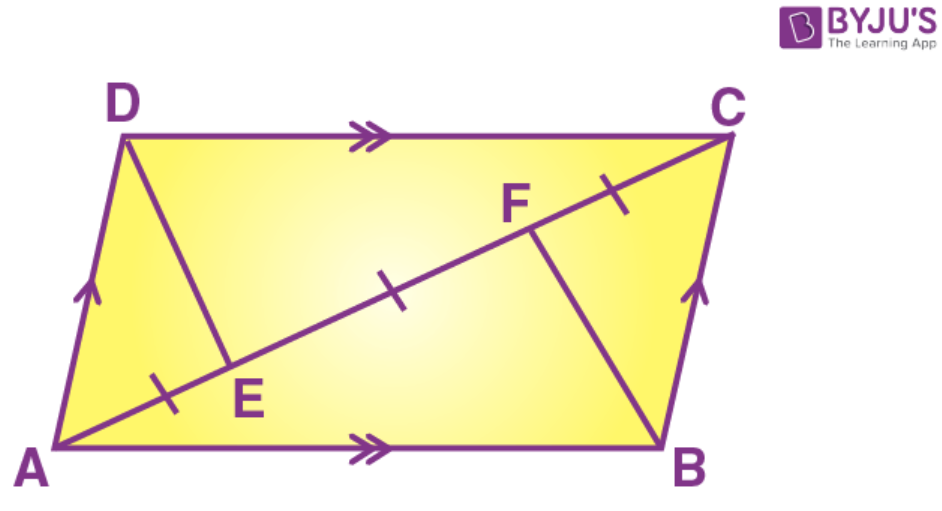Solution:

Construction:

Join DF and EB

Join diagonal BD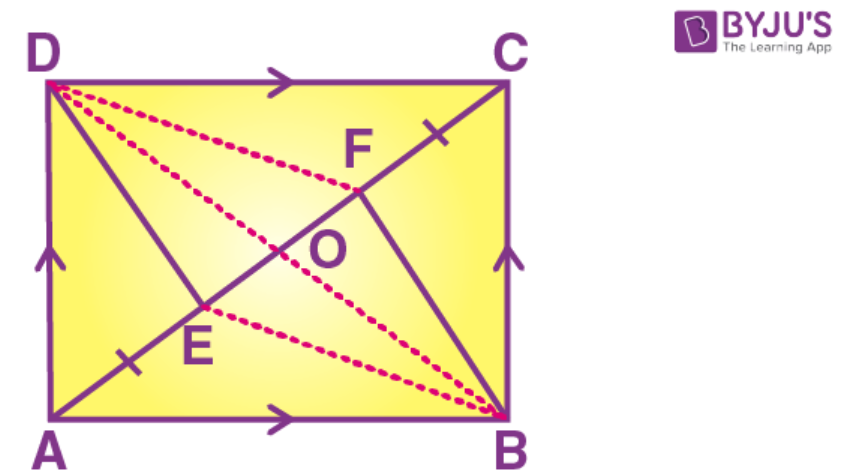Since the diagonals of a parallelogram bisect each other

Therefore, OA = OC and OB = OD

Also, AE = EF = FC

Now, OA = OC and AE = FC

OA – OC = OC – FC

OE = OF

Thus, in quadrilateral DEFB, we have

OB = OD and OE = OF

Diagonals of a quadrilateral DEFB bisect each other.

DEFB is a parallelogram

DE is parallel to FB

DE = FB (opposite sides are equal)

4. In the alongside diagram, ABCD is a parallelogram in which AP bisects angle AP bisects angle A and BQ bisects angle B. Prove that: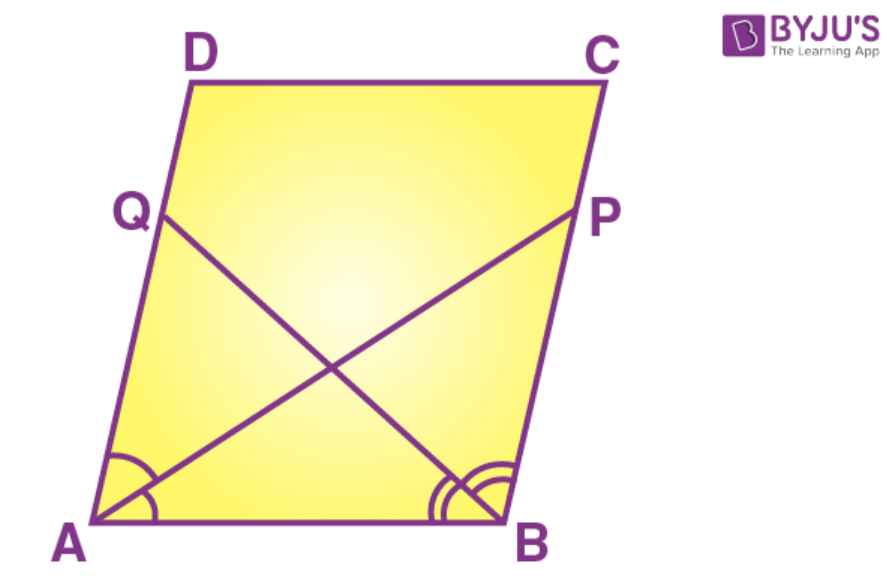(i) AQ = BP

(ii) PQ = CD.

(iii) ABPQ is a parallelogram.

Solution: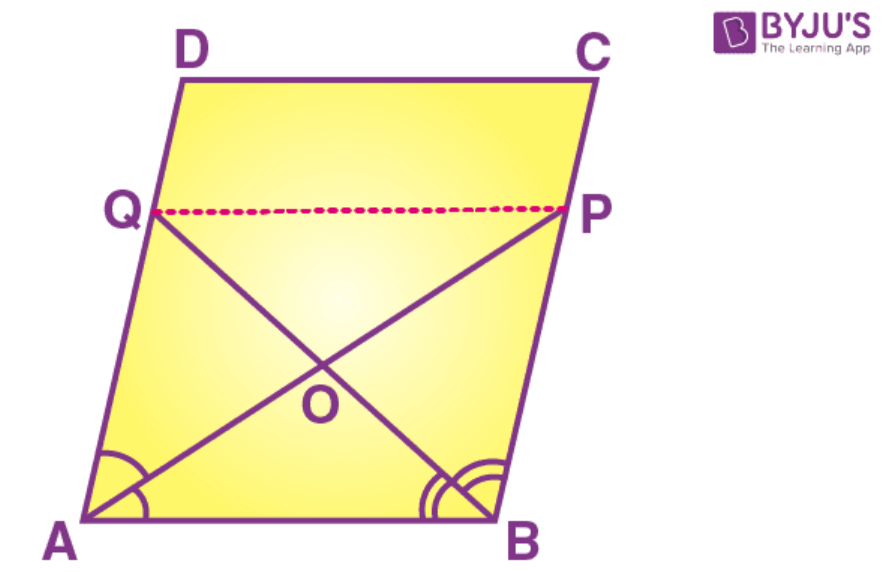Consider the triangle AOQ and triangle BOP

∠AOQ = ∠BOP [opposite angles]

∠OAQ = ∠BPO [alternate angles]

△ AOQ △ BOP

Hence AQ = BP

Consider the triangle QOP and triangle AOB

∠AOB = ∠QOP [opposite angles]

∠OAB = ∠APQ [alternate angles]

△ QOP △ AOB

Hence PQ = AB = CD

DQ = CP and DQ parallel to CP

Also, QP = DC and AB parallel to QP parallel to DC

Hence quadrilateral QPCD is a parallelogram.

5. In the given figure, ABCD is a parallelogram. Prove that: AB = 2 BC.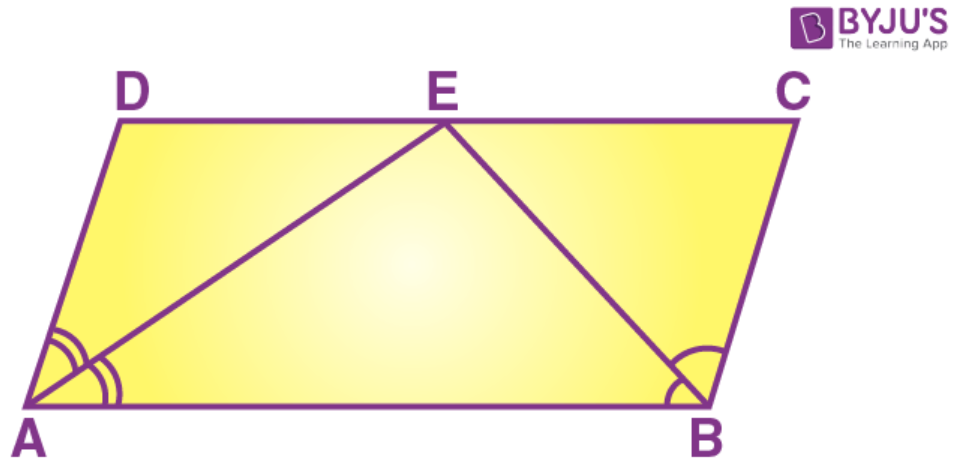Solution:

Given: ABCD is a parallelogram

To prove: AB = 2BC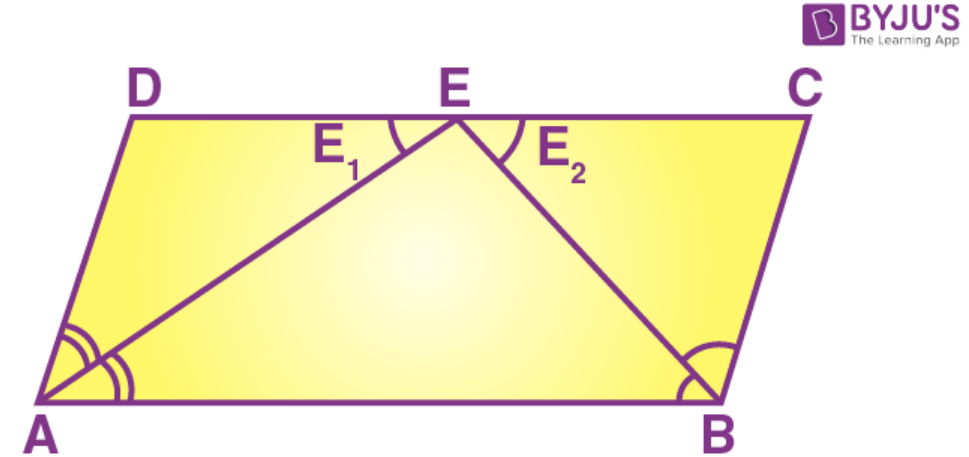Proof: ABCD is a parallelogram

∠A + ∠D = ∠B + ∠C = 180o

From the triangle AEB we have

∠A/2 + ∠B/2 + ∠E = 180o

∠A – ∠A/2 + ∠D + ∠E1= 180o [taking E1 as a new angle]

∠A + ∠D + ∠E1 = 180o + ∠A/2

∠E1 = ∠A/2 [since ∠A + ∠D = 180o]

Again, similarly

∠E2 = ∠B/2

Now,

AB = DE + EC

= 2 BC [since AD = BC]

## Selina Solutions for Class 9 Maths Chapter 14- Rectilinear figures

The Chapter 14, Rectilinear figures, contains 3 exercises and the Solutions given here includes the answers for all the questions present in these exercises. Let us have a look at some of the topics that are being discussed in this chapter.

14.1 Introduction

14.2 Names of polygon

14.3 Regular Polygon

1. Trapezium
2. Parallelogram
3. Rectangle
4. Rhombus
5. Square

14.6 Diagonal properties

1. In a parallelogram, both the pairs of opposite sides are equal.
2. In a parallelogram, both the pairs of opposite angles are equal
3. If one pair of opposite sides of a quadrilateral are equal and parallel, it is a parallelogram.
4. Each diagonal of a parallelogram bisects the parallelogram
5. The diagonals of a parallelogram bisect each other.
6. Rhombus is a special parallelogram whose diagonals meet at right angles.
7. In a rectangle, diagonals are equal.
8. In a square, diagonals are equal and meet at right angles.

## Selina Solutions for Class 9 Maths Chapter 14- Rectilinear figures

In Chapter 14 of Class 9, the students are taught about Rectilinear figures or quadrilaterals. The chapter discusses different properties and theorems related to quadrilaterals like squares, parallelograms, trapezium, rectangle etc. The chapter helps the students in understanding Rectilinear figures. Study the Chapter 14 of Selina textbook to understand more about Rectilinear figures. Learn the Selina Solutions for Class 9 effectively to come out with excellent marks in the examinations.